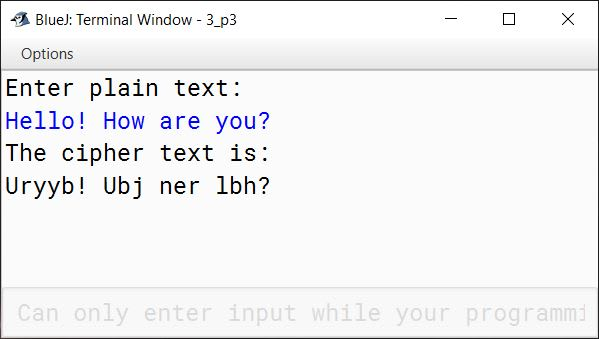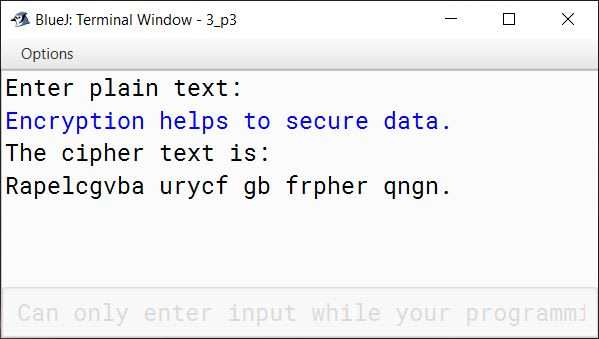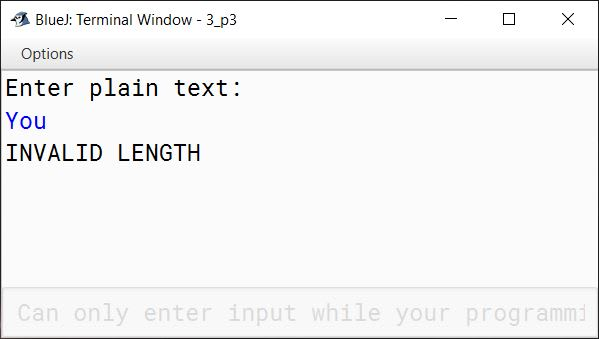# String Manipulations

## Solutions to Unsolved Programs

#### Question 1

A string is given as:
Purity of Mind is Essential

Write a program in Java to enter the string. Count and display:

1. The character with lowest ASCII code in lower case
2. The character with highest ASCII code in lower case
3. The character with lowest ASCII code in upper case
4. The character with highest ASCII code in upper case

Sample Output:

The character with lowest ASCII code in lower case: a
The character with highest ASCII code in lower case: y
The character with lowest ASCII code in upper case: E
The character with highest ASCII code in upper case: P

Solution

```import java.util.Scanner;

public class KboatASCIICode
{
public static void main(String args[]) {
Scanner in = new Scanner(System.in);
System.out.println("Enter the string:");
String str = in.nextLine();
int len = str.length();

/*
* Intialize low variables to
* highest ASCII code and
* high variables to lowest
* ASCII code
*/
char lowerCaseLow = 255;
char lowerCaseHigh = 0;
char upperCaseLow = 255;
char upperCaseHigh = 0;

for (int i = 0; i < len; i++) {
char ch = str.charAt(i);
if (Character.isLowerCase(ch)) {
if (ch < lowerCaseLow) {
lowerCaseLow = ch;
}

if (ch > lowerCaseHigh) {
lowerCaseHigh = ch;
}
}
else if (Character.isUpperCase(ch)) {
if (ch < upperCaseLow) {
upperCaseLow = ch;
}

if (ch > upperCaseHigh) {
upperCaseHigh = ch;
}
}
}

System.out.println("The character with lowest ASCII code in lower case: "
+ lowerCaseLow);
System.out.println("The character with highest ASCII code in lower case: "
+ lowerCaseHigh);
System.out.println("The character with lowest ASCII code in upper case: "
+ upperCaseLow);
System.out.println("The character with highest ASCII code in upper case: "
+ upperCaseHigh);
}
}```
##### Output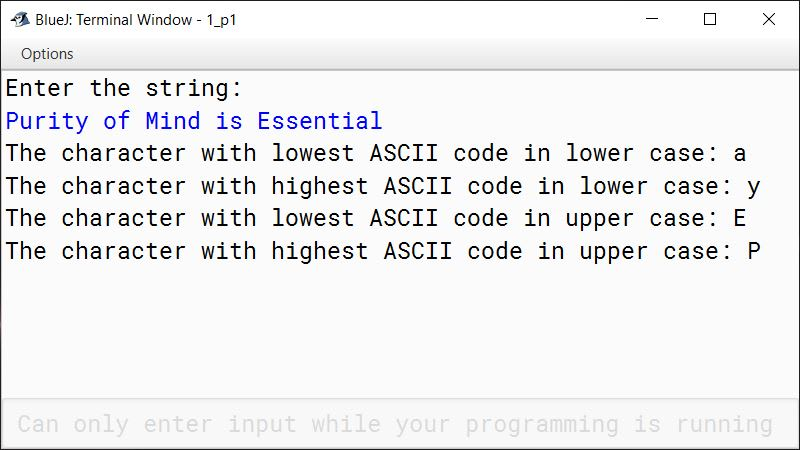#### Question 2

Write a program in Java to enter a string in a mixed case. Arrange all the letters of string such that all the lower case characters are followed by the upper case characters.

Sample Input:
Computer Science

Sample Output:
omputercienceCS

Solution

```import java.util.Scanner;

public class KboatStringLowerThenUpper
{
public static void main(String args[]) {
Scanner in = new Scanner(System.in);
System.out.print("Enter string: ");
String str = in.nextLine();
int len = str.length();
StringBuffer sbLowerCase = new StringBuffer();
StringBuffer sbUpperCase = new StringBuffer();

for (int i = 0; i < len; i++) {
char ch = str.charAt(i);
if (Character.isLowerCase(ch))
sbLowerCase.append(ch);
else if (Character.isUpperCase(ch))
sbUpperCase.append(ch);
}

System.out.println("Input String:");
System.out.println(str);
String newStr = sbLowerCase.append(sbUpperCase).toString();
System.out.println("Changed String:");
System.out.print(newStr);
}
}```
##### Output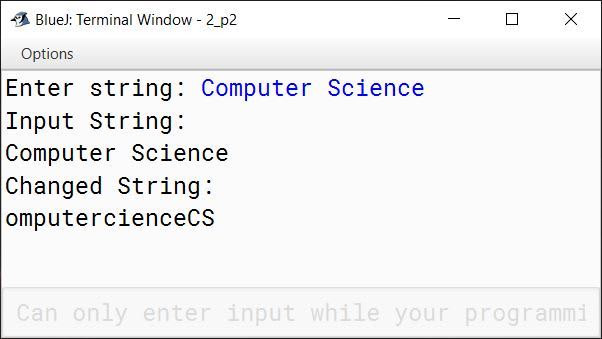#### Question 3

Write a program in Java to accept a string. Arrange all the letters of the string in an alphabetical order. Now, insert the missing letters in the sorted string to complete all the letters between first and last characters of the string.

Sample Input:
computer

Alphabetical order:
cemoprtu

Sample Output:
cdefghijklmnopqrstu

Solution

```import java.util.Scanner;

public class KboatStringSort
{
public static void main(String args[]) {
Scanner in = new Scanner(System.in);
System.out.println("Enter a string:");
String str = in.nextLine();
str = str.toLowerCase();
int len = str.length();

String sortedStr = ""; //Empty String
for (char ch = 'a'; ch <= 'z'; ch++) {
for (int i = 0; i < len; i++) {
char strCh = str.charAt(i);
if (ch == strCh) {
sortedStr += strCh;
}
}
}

System.out.println("Alphabetical order:");
System.out.println(sortedStr);

String filledStr = ""; //Empty String
for (int i = 0; i < len - 1; i++) {
char strCh = sortedStr.charAt(i);
filledStr += strCh;
for (int j = strCh + 1; j < sortedStr.charAt(i+1); j++) {
filledStr += (char)j;
}
}
filledStr += sortedStr.charAt(len - 1);

System.out.println("Filled String:");
System.out.println(filledStr);
}
}```
##### Output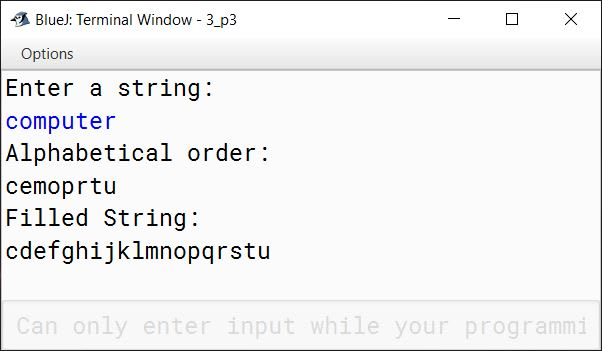#### Question 4

Write a program in Java to accept a string. Count and display the frequency of each character present in the string. The character with multiple frequencies should be displayed only once.

Sample Input:
golden jubilee

Sample Output:

 Alphabet g o l d e n j u b i Frequency 1 1 2 1 3 1 1 1 1 1

Solution

```import java.util.Scanner;

public class KboatStringFreq
{
public static void main(String args[]) {
Scanner in = new Scanner(System.in);
System.out.println("Enter a string:");
String str = in.nextLine();
int len = str.length();
char seenArr[] = new char[len];
int freqArr[] = new int[len];
int idx = 0;

for (int i = 0; i < len; i++) {
char ch = str.charAt(i);

if (Character.isWhitespace(ch)) {
continue;
}

boolean seen = false;
for (int j = 0; j < idx; j++) {
if (ch == seenArr[j]) {
seen = true;
break;
}
}

if (seen) {
continue;
}

int f = 1;
for (int k = i + 1; k < len; k++) {
if (ch == str.charAt(k)) {
f++;
}
}

seenArr[idx] = ch;
freqArr[idx] = f;
idx++;
}

for (int i = 0; i < idx; i++) {
System.out.print(seenArr[i] + " ");
}

System.out.println();
for (int i = 0; i < idx; i++) {
System.out.print(freqArr[i] + " ");
}
}
}```
##### Output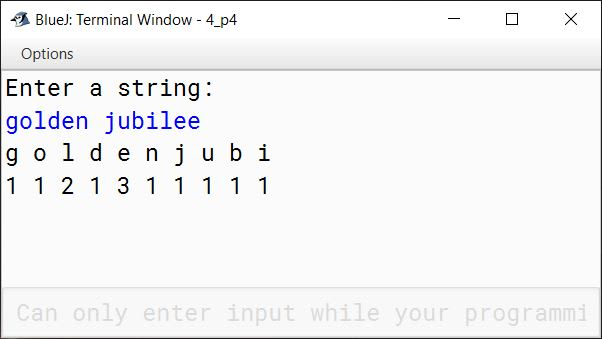#### Question 5

Write a program in Java to accept a string and display the new string after reversing the characters of each word.

Sample Input:
Understanding Computer Science

Sample output:
gnidnatsrednU retupmoC ecneicS

Solution

```import java.util.*;

public class KboatReverse
{
public static void main(String args[]) {
Scanner in = new Scanner(System.in);
System.out.println("Enter a string:");
String str = in.nextLine();
int len = str.length();
String revStr = ""; //Empty String

StringTokenizer st = new StringTokenizer(str);
while (st.hasMoreTokens()) {
String word = st.nextToken();
int wordLen = word.length();
for (int i = wordLen - 1; i >= 0; i--) {
revStr += word.charAt(i);
}
revStr += " ";
}

System.out.println("String with words reversed:");
System.out.println(revStr);
}
}```
##### Output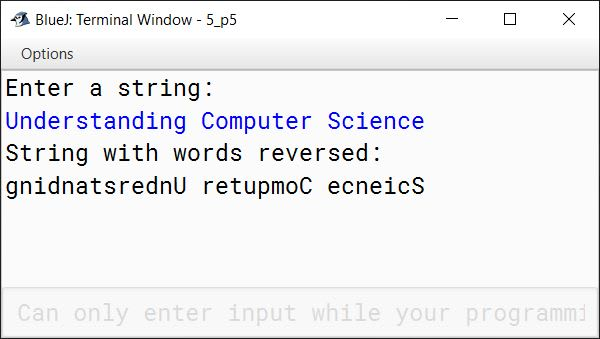#### Question 6

Write a program in Java to accept two strings. Display the new string by taking each character of the first string from left to right and of the second string from right to left. The letters should be taken alternatively from each string. Assume that the length of both the strings are same.

Sample Input:
String 1: HISTORY
String 2: SCIENCE

Sample Output:
HEICSNTEOIRCYS

Solution

```import java.util.Scanner;

public class KboatString
{
public static void main(String args[]) {
Scanner in = new Scanner(System.in);
System.out.print("Enter first String: ");
String s1 = in.nextLine();
System.out.print("Enter second String: ");
String s2 = in.nextLine();
String newStr = "";
int len = s1.length();

for (int i = 0; i < len; i++) {
char ch1 = s1.charAt(i);
char ch2 = s2.charAt(len - 1 - i);
newStr = newStr + ch1 + ch2;
}

System.out.println(newStr);
}
}```
##### Output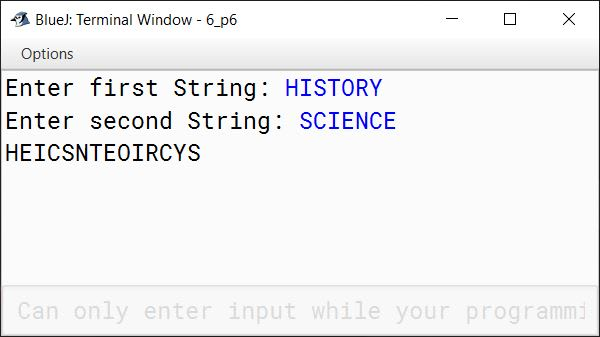#### Question 7

Write a program in Java to accept a four-letter word. Display all the probable four letter combinations such that no letter should be repeated in the output within each combination.

Sample Input:
PARK

Sample Output:
PAKR, PKAR, PRAK, APRK, ARPK, AKPR, and so on.

Solution

```import java.util.Scanner;

public class KboatStringCombinations
{
public static void main(String args[]) {
Scanner in = new Scanner(System.in);
System.out.println("Enter a word:");
String str = in.nextLine();
int len = str.length();

if (len != 4) {
System.out.println("Invalid Input!");
System.out.println("Please enter a four letter word");
return;
}

for (int i = 0; i < len; i++) {
for (int j = 0; j < len; j++) {
for (int k = 0; k < len; k++) {
for (int l = 0; l < len; l++) {
if (i != j && i != k && i != l
&& j != k && j != l
&& k != l) {
System.out.print(str.charAt(i));
System.out.print(str.charAt(j));
System.out.print(str.charAt(k));
System.out.println(str.charAt(l));
}
}
}
}
}
}
}```
##### Output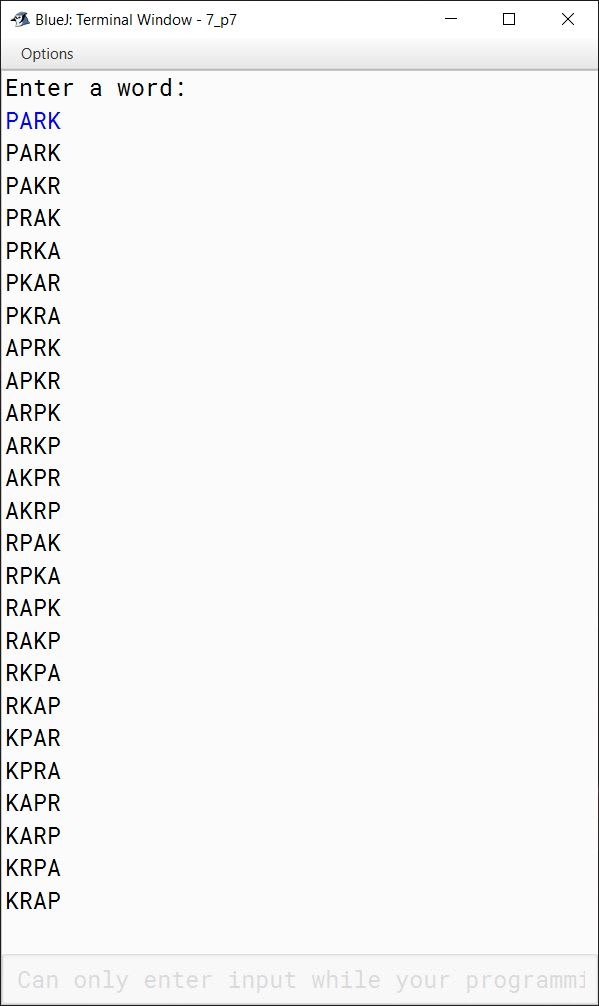#### Question 8

While typing, a typist has created two or more consecutive blank spaces between the words of a sentence. Write a program in Java to eliminate multiple blanks between the words by a single blank.

Sample Input:
Indian   Cricket   team   tour   to   Australia

Sample Output:
Indian Cricket team tour to Australia

Solution

```import java.util.*;

public class KboatMultipleBlanks
{
public static void main(String args[]) {
Scanner in = new Scanner(System.in);
System.out.println("Enter a string:");
String str = in.nextLine();
int len = str.length();

StringTokenizer st = new StringTokenizer(str);
String newStr = ""; //Empty String

while (st.hasMoreTokens()) {
String word = st.nextToken();
newStr += word + " ";
}

newStr = newStr.trim();

System.out.println("Output String:");
System.out.println(newStr);
}
}```
##### Output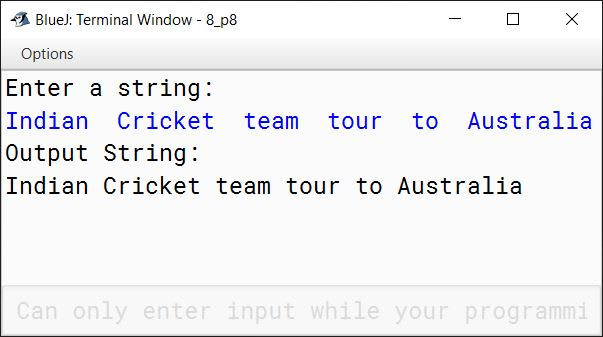#### Question 9

A new advanced Operating System, incorporating the latest hi-tech features has been designed by Opera Computer Systems. The task of generating copy protection code to prevent software privacy has been entrusted to the Security Department. The Security Department has decided to have codes containing a jumbled combination of alternate uppercase letters of the alphabet starting from A up to K (namely among A, C, E, G, I, K). The code may or may not be in the consecutive series of alphabets. Each code should not exceed 6 characters and there should be no repetition of characters. If it exceeds 6 characters, display an appropriate error message.

Write a program to input a code and its length. At the first instance of an error display "Invalid" stating the appropriate reason. In case of no error, display the message "Valid".

Sample Data:

Input:
n=4
ABCE

Output:
Invalid! Only alternate letters permitted!

Input:
n=4
AcIK

Output:
Invalid! Only uppercase letters permitted!

Input:
n = 7

Output:
Error! Length of String should not exceed 6 characters!

Input:
n=3
ACE

Output:
Valid

Input:
n=5
GEAIK

Output:
Valid

Solution

```import java.util.Scanner;

public class KboatCodeCheck
{
public static void main(String args[]) {
Scanner in = new Scanner(System.in);
System.out.print("Enter length: ");
int len = in.nextInt();
in.nextLine();

if (len < 1 || len > 6) {
System.out.println("Error! Length of String should not exceed 6 characters!");
return;
}

System.out.println("Enter Code:");
String code = in.nextLine();

for (int i = 0; i < len; i++) {
char ch = code.charAt(i);

if (Character.isLowerCase(ch)) {
System.out.println("Invalid! Only uppercase letters permitted!");
return;
}

if (ch < 'A' || ch > 'K') {
System.out.println("Invalid! Only letters between A and K are permitted!");
return;
}

/*
* ASCII Code should be odd as we
* are taking alternate letters
* between A and K. We have already
* checked above that letter is
* between A and K. Now if we check
* for odd then it means that letter
* is one of A, C, E, G, I, K
*/

if (ch % 2 == 0) {
System.out.println("Invalid! Only alternate letters permitted!");
return;
}

/*
* Check for repetition
*/

int count = 0;
for (int j = 0; j < len; j++) {
if (ch == code.charAt(j)) {
count++;
}
}

if (count > 1) {
System.out.println("Invalid! Letter repetition is not permitted!");
return;
}
}

System.out.println("Valid");
}
}```
##### Output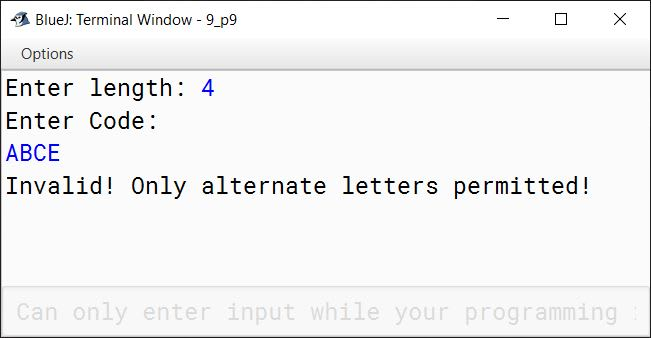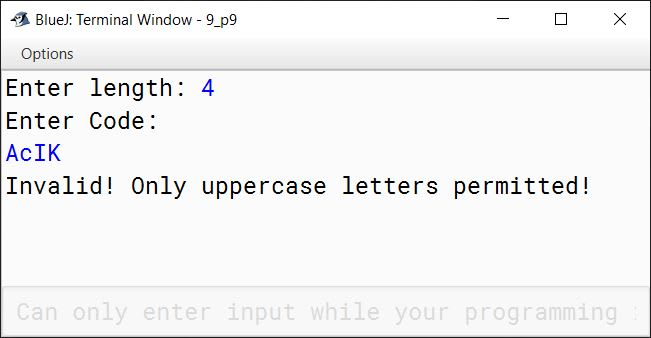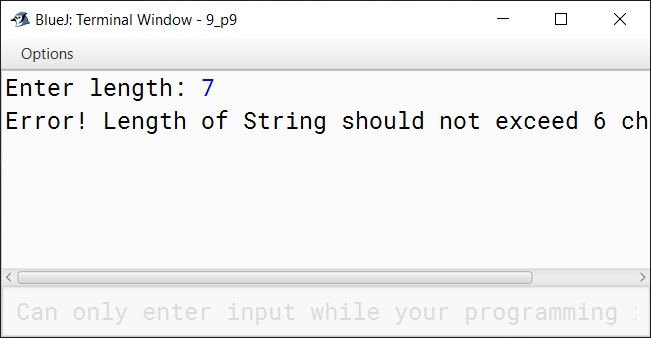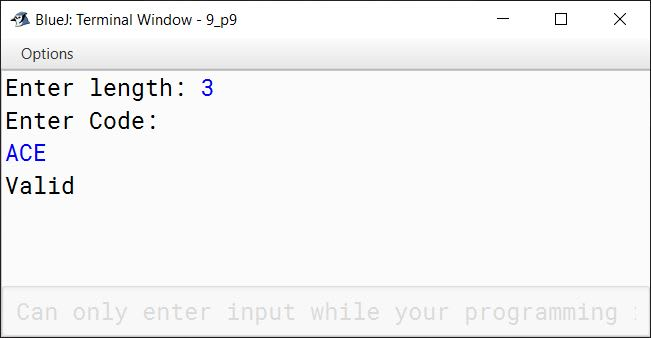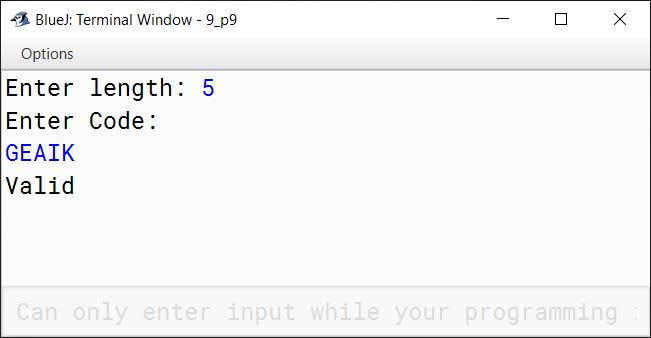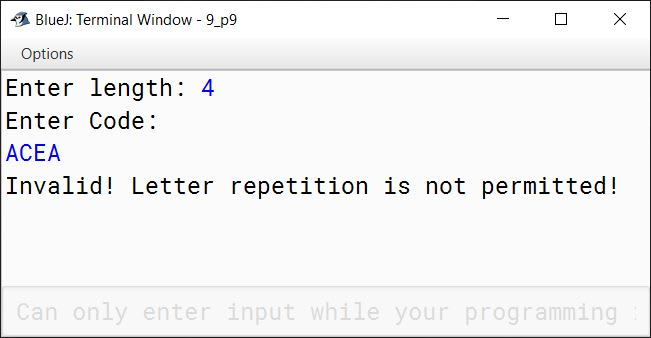#### Question 10

The input in this question will consist of a number of lines of English text consisting of the letters of the English alphabet, the punctuation marks (') apostrophe, (.) full stop (, ) comma, (; ) semicolon, (:) colon and white space characters (blank, new line). Your task is to print the words of the text in reverse order without any punctuation marks other than blanks.

For example, consider the following input text:

This is a sample piece of text to illustrate this question.
If you are smart you will solve this right.

The corresponding output would read as:

right this solve will you smart are you if question this illustrate to text of piece sample a is this

Note: Individual words are not reversed.

Input Format:

This first line of input contains a single integer n ( < = 20), indicating the number of lines in the input.
This is followed by lines of input text. Each line should accept a maximum of 80 characters.

Output Format:

Output the text containing the input lines in reverse order without punctuations except blanks as illustrated above.

Test your program for the following data and some random data.

Sample Data:

Input:
2
Emotions controlled and directed to work, is character.
By Swami Vivekananda.

Output:
Vivekananda Swami By character is work to directed and controlled Emotions

Input:
1
Do not judge a book by its cover.

Output:
cover its by book a judge not Do

Solution

```import java.util.*;

public class KboatTextReverse
{
public static void main(String args[]) {
Scanner in = new Scanner(System.in);
System.out.print("Enter number of lines (n): ");
int n = in.nextInt();
in.nextLine();

if (n < 1 || n > 20) {
System.out.println("Invalid number of lines");
return;
}

String punctuations = "'.,;:";

System.out.println("Enter Lines:");
String lines[] = new String[n];
for (int i = 0; i < n; i++) {
lines[i] = in.nextLine();
if (lines[i].length() > 80) {
System.out.println("Invalid line!");
System.out.println("Length should be within 80 characters");
return;
}
}

StringBuffer sbLine = new StringBuffer();
for (int i = 0; i < n; i++) {

StringTokenizer st = new StringTokenizer(lines[i]);

while (st.hasMoreTokens()) {
StringBuffer sbWord = new StringBuffer();
String word = st.nextToken();
int len = word.length();
for (int j = 0; j < len; j++) {
char ch = word.charAt(j);
if (!Character.isWhitespace(ch)
&& punctuations.indexOf(ch) == -1) {
sbWord.append(ch);
}
}
sbLine.insert(0, sbWord.toString());
sbLine.insert(0, " ");
}
}

String reversedLine = sbLine.toString().trim();
System.out.println(reversedLine);

}
}```
##### Output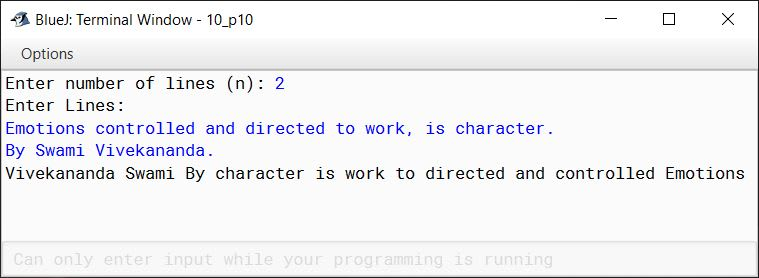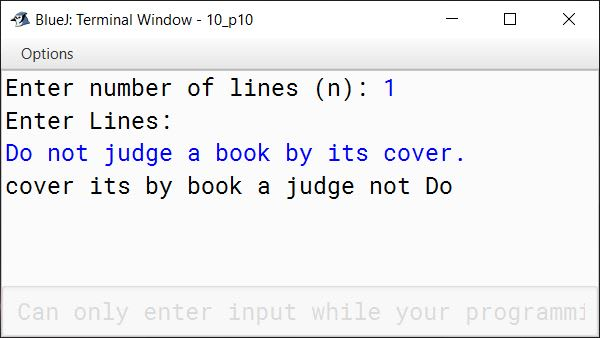#### Question 11

Read a single sentence which terminates with a full stop (.). The words are to be separated with a single blank space and are in lower case. Arrange the words contained in the sentence according to the length of the words in ascending order. If two words are of the same length then the word occurring first in the input sentence should come first. For both, input and output the sentence must begin in upper case.

Test your program for given data and also some random data:

Input:
The lines are printed in reverse order.
Output:
In the are lines order printed reverse.

Input:
Print the sentence in ascending order.
Output:
In the print order sentence ascending.

Input:
I love my Country.
Output:
I my love Country.

Solution

```import java.util.*;

public class KboatWordLenSort
{
public static void main(String args[]) {
Scanner in = new Scanner(System.in);
System.out.println("Enter a sentence:");
String str = in.nextLine();
int len = str.length();

if (str.charAt(len - 1) != '.') {
System.out.println("Invalid Input!");
System.out.println("Sentence should end with full stop.");
return;
}

if (Character.isLowerCase(str.charAt(0))) {
System.out.println("Invalid Input!");
return;
}

String ipStr = Character.toLowerCase(str.charAt(0)) + str.substring(1, len - 1);
StringTokenizer st = new StringTokenizer(ipStr);
int wordCount = st.countTokens();
String strArr[] = new String[wordCount];

for (int i = 0; i < wordCount; i++) {
strArr[i] = st.nextToken();
}

for (int i = 0; i < wordCount - 1; i++) {
for (int j = 0; j < wordCount - i - 1; j++) {
if (strArr[j].length() > strArr[j + 1].length()) {
String t = strArr[j];
strArr[j] = strArr[j+1];
strArr[j+1] = t;
}
}
}

strArr = Character.toUpperCase(strArr.charAt(0))
+ strArr.substring(1);

System.out.println("Sorted String:");
for (int i = 0; i < wordCount; i++) {
System.out.print(strArr[i]);
if (i == wordCount - 1) {
System.out.print(".");
}
else {
System.out.print(" ");
}
}
}
}```
##### Output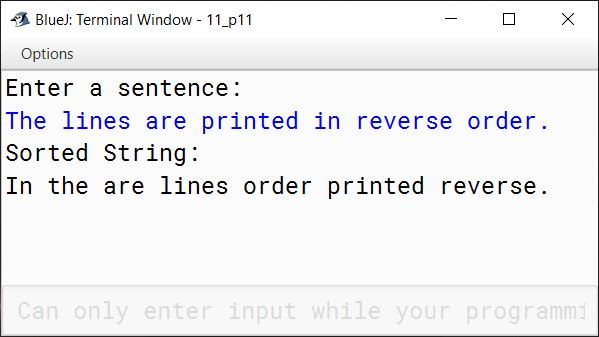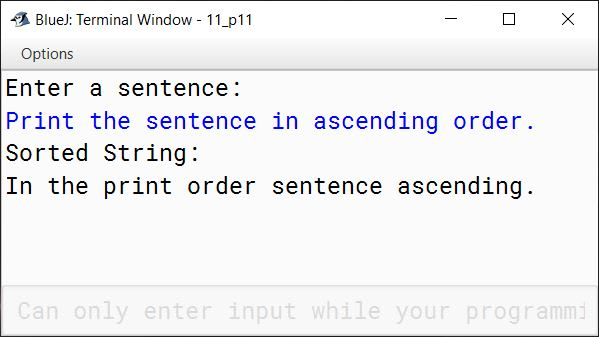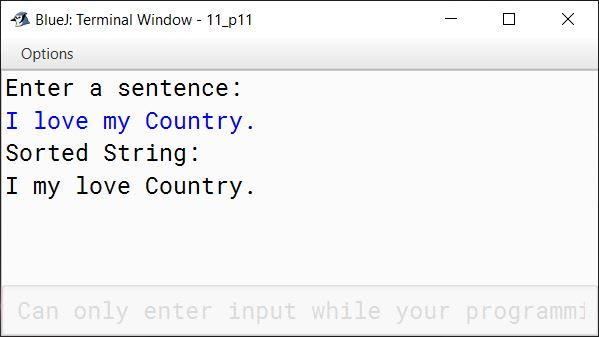#### Question 12

Write a program to read a string / sentence and output the number of times each word occurs in the entire text. At the end, the output should be sorted into ascending order words along with usage of words. You may assume that the entire text is in capitals (you may also convert in capitals for your betterment) and each word is followed by a blank space except the last one, which is followed by a full stop. Let there be at the most 50 words in the text.

Test your program for the given sample data and also some other random data:

SAMPLE DATA:

Input:
YOU ARE GOOD WHEN YOUR THOUGHTS ARE GOOD AND YOUR DEEDS ARE GOOD.

Output:

WordsWord Count
And1
Are3
Deeds1
Good3
Thoughts1
When1
You1
Your2

Input:
IF YOU FAIL TO PLAN YOU ARE PLANNING TO FAIL.

Output:

WordsWord Count
Are1
Fail2
If1
Plan1
Planning1
To2
You2

Solution

```import java.util.*;

public class KboatWordFreq
{
public static void main(String args[]) {
Scanner in = new Scanner(System.in);
System.out.println("Enter a string:");
String str = in.nextLine();
str = str.toUpperCase();
int len = str.length();

StringTokenizer st = new StringTokenizer(str.substring(0, len - 1));
int wordCount = st.countTokens();
String strArr[] = new String[wordCount];

if (wordCount > 50) {
System.out.println("Invalid Input!");
System.out.println("Number of words should be upto 50 only.");
return;
}

for (int i = 0; i < wordCount; i++) {
strArr[i] = st.nextToken();
}

for (int i = 0; i < wordCount - 1; i++) {
for (int j = 0; j < wordCount - i - 1; j++) {
if (strArr[j].compareTo(strArr[j+1]) > 0) {
String t = strArr[j];
strArr[j] = strArr[j+1];
strArr[j+1] = t;
}
}
}

System.out.println(); //To Print a blank line
System.out.println("Word\t\tWord Count");
int count = 0;
for (int i = 0; i < wordCount - 1; i++) {
count++;
if (!strArr[i].equals(strArr[i + 1])) {
System.out.println(strArr[i] + "\t\t" + count);
count = 0;
}
}

//Print last word of array
count++;
System.out.println(strArr[wordCount - 1] + "\t\t" + count);
}
}```
##### Output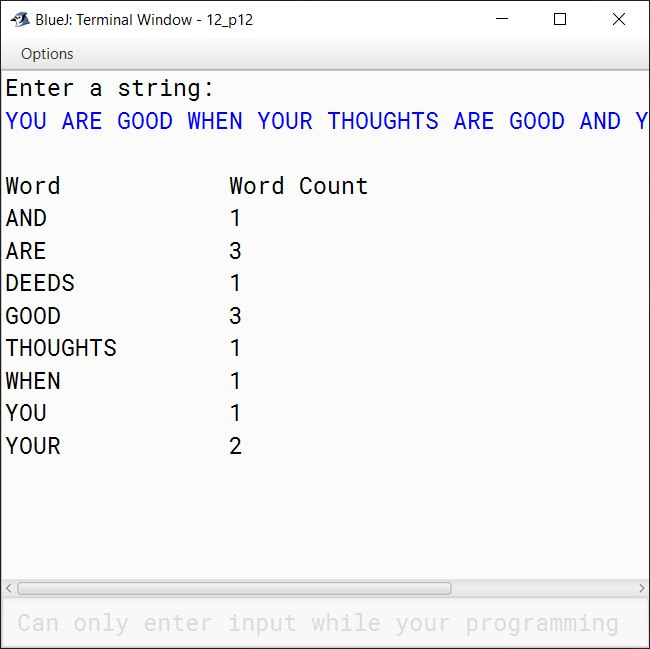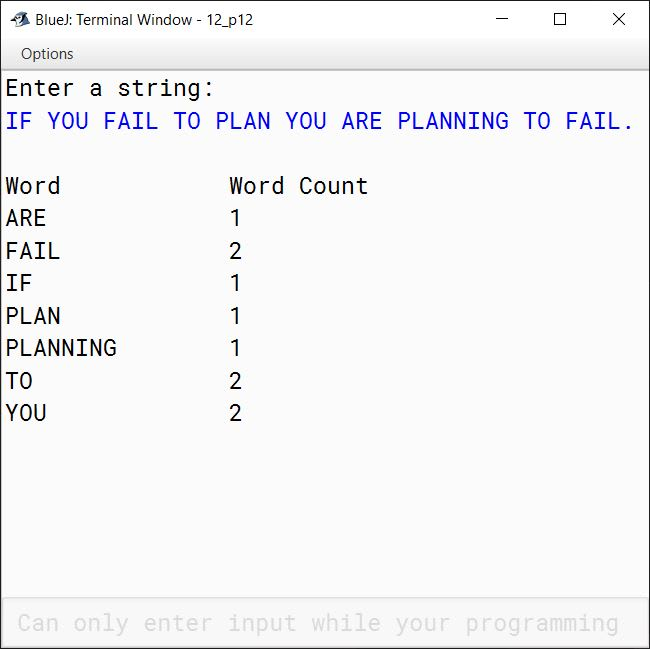#### Question 13

A string is said to be valid if it contains pair of parenthesis having text / alphabet such as (TY) and the string is said to be invalid if it contains nested parenthesis such as (Y (UP)).

For example:
SUN (A(X) RISE) BEGINS FROM (RT) EAST is an "Invalid" string because in this string nested parenthesis are present, but SUN (A) RISE BEGINS FROM (RT) EAST is a "Valid" string because there is no nested parenthesis.

Write a program to:

1. Read a string / sentence and convert in capitals.
2. Check the validity of the given string.
3. If the string is valid, output the given string omitting the portion enclosed in brackets otherwise, output a message "Sorry, and invalid string".

Test your program for the given sample data and also some other random data:

Sample Data:

Input:
SUN (a) RISE BEGINS FROM (RT) EAST
Output:
SUN RISE BEGINS FROM EAST

Input:
SUN (A (X) RISE) BEGINS FROM (RT) EAST
Output:
Sorry, an invalid string

Input:
COM(IPX)PUTER IS (MY) JUNK (GOOD) SYSTEM
Output:
COMPUTER IS JUNK SYSTEM

Solution

```import java.util.*;

public class KboatParenthesisCheck
{
public static void main(String args[]) {
Scanner in = new Scanner(System.in);
System.out.println("Enter a string:");
String str = in.nextLine();
str = str.toUpperCase();
int len = str.length();

StringBuffer sb = new StringBuffer();

int open = -1;  //Contains index of opening parenthesis

for (int i = 0; i < len; i++) {
char ch = str.charAt(i);
if (ch == '(') {
if (open != -1) {
System.out.println("Sorry, an invalid string");
return;
}

open = i;
}

if (open == -1) {
sb.append(ch);
}

if (ch == ')') {
if (open == -1) {
System.out.println("Sorry, an invalid string");
return;
}

open = -1;
}

}

/*
* Using StringTokenizer to remove
* extra spaces between words
*/

StringTokenizer st = new StringTokenizer(sb.toString());
while (st.hasMoreTokens()) {
System.out.print(st.nextToken());
System.out.print(" ");
}

}
}```
##### Output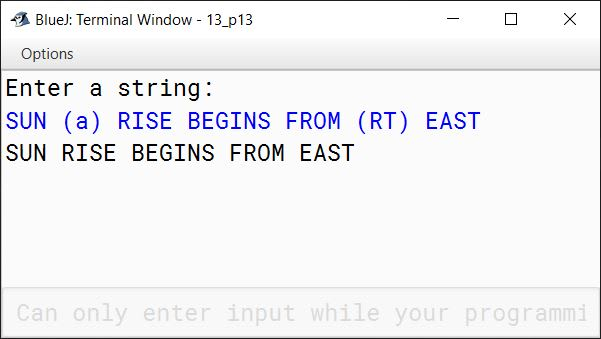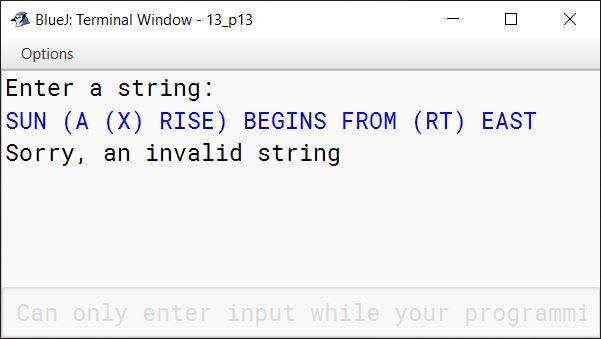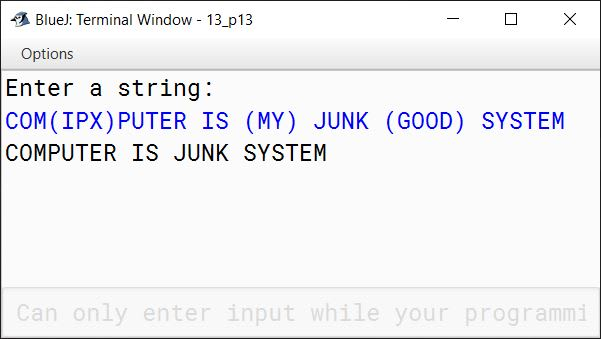#### Question 14

Input a paragraph containing 'n' number of sentences where (1 < = n < 4). The words are to be separated with a single blank space and are in UPPERCASE. A sentence may be terminated either with a full stop '.' Or a question mark '?' only. Any other character may be ignored. Perform the following operations:

1. Accept the number of sentences. If the number of sentences exceeds the limit, an appropriate error message must be displayed.
2. Find the number of words in the whole paragraph.
3. Display the words in ascending order of their frequency. Words with same frequency may appear in any order.

Example 1

Input:
Enter number of sentences.
1
Enter sentences.
TO BE OR NOT TO BE.

Output:
Total number of words: 6

WordFrequency
OR1
NOT1
TO2
BE2

Example 2

Input:
Enter number of sentences.
3
Enter sentences.
THIS IS A STRING PROGRAM. IS THIS EASY? YES IT IS.

Output:
Total number of words: 11

WordFrequency
A1
STRING1
PROGRAM1
EASY1
YES1
IT1
THIS2
IS3

Example 3

Input:
Enter number of sentences. 5

Output:
Invalid Entry

Solution

```import java.util.*;

public class KboatPara
{
public static void main(String args[]) {
Scanner in = new Scanner(System.in);
System.out.println("Enter number of sentences.");
int n = in.nextInt();
in.nextLine();

if (n < 1 || n > 3) {
System.out.println("Invalid Entry");
return;
}

System.out.println("Enter sentences.");
String ipStr = in.nextLine();
ipStr = ipStr.toUpperCase();

StringTokenizer st = new StringTokenizer(ipStr, " .?");
int wordCount = st.countTokens();

System.out.println();
System.out.println("Total number of words: " + wordCount);

String wordArr[] = new String[wordCount];
int wordFreq[] = new int[wordCount];
int idx = 0;

for (int i = 0; i < wordCount; i++) {

String word = st.nextToken();
int j = 0;

for (j = 0; j < idx; j++) {
if (wordArr[j].equals(word)) {
wordFreq[j]++;
break;
}
}

if (j == idx) {
wordArr[idx] = word;
wordFreq[idx]++;
idx++;
}
}

//Sort Word Frequency in ascending order
for (int i = 0; i < idx - 1; i++) {
for (int j = 0; j < idx - i - 1; j++) {
if (wordFreq[j] > wordFreq[j + 1]) {
int t = wordFreq[j];
wordFreq[j] = wordFreq[j+1];
wordFreq[j+1] = t;

String temp = wordArr[j];
wordArr[j] = wordArr[j+1];
wordArr[j+1] = temp;
}
}
}

//Display the words and frequencies
System.out.println("Word\tFrequency");
for (int i = 0; i < idx; i++) {
System.out.println(wordArr[i] + "\t" + wordFreq[i]);
}
}
}```
##### Output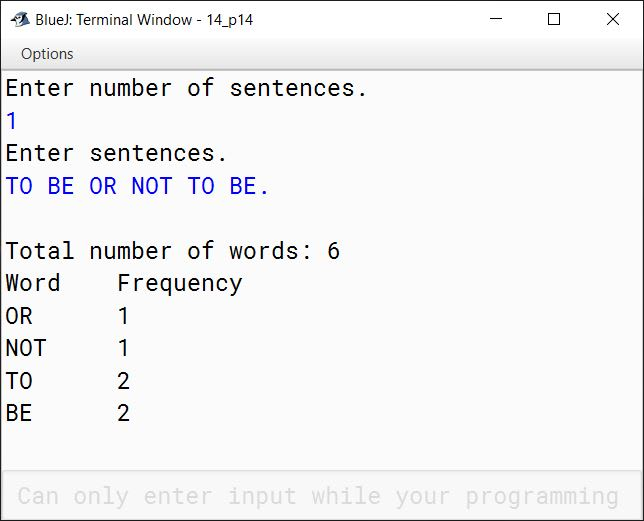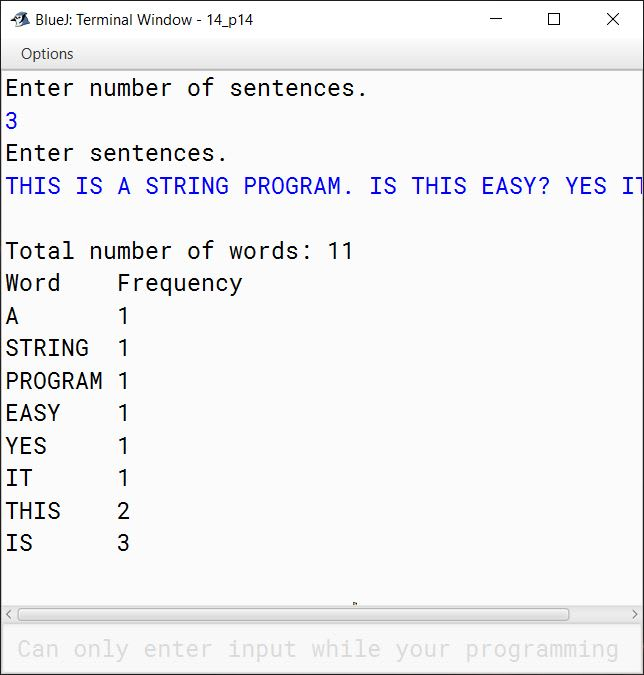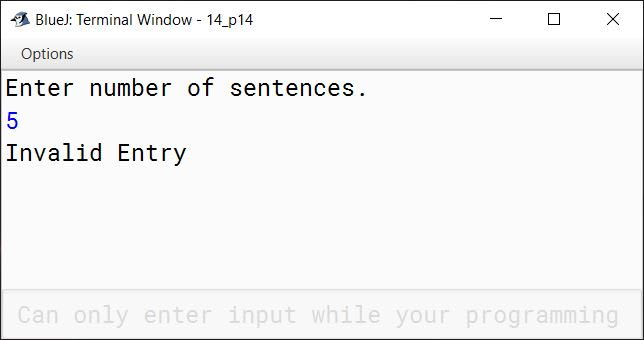#### Question 15

Caesar Cipher is an encryption technique which is implemented as ROT13 ('rotate by 13 places'). It is a simple letter substitution cipher that replaces a letter with the letter 13 places after it in the alphabets, with the other characters remaining unchanged.

ROT13

 A/a B/b C/c D/d E/e F/f G/g H/h I/i J/j K/k L/l M/m ↕ ↕ ↕ ↕ ↕ ↕ ↕ ↕ ↕ ↕ ↕ ↕ ↕ N/n O/o P/p Q/q R/r S/s T/t U/u V/v W/w X/x Y/y Z/z

Write a program to accept a plain text of length L, where L must be greater than 3 and less than 100.

Encrypt the text if valid as per the Caesar Cipher.

Test your program with the sample data and some random data.

Example 1

INPUT:
Hello! How are you?

OUTPUT:
The cipher text is:
Uryyb! Ubj ner lbh?

Example 2

INPUT:
Encryption helps to secure data.

OUTPUT:
The cipher text is:
Rapelcgvba urycf gb frpher qngn.

Example 3

INPUT:
You

OUTPUT:
INVALID LENGTH

Solution

```import java.util.Scanner;

public class CaesarCipher
{
public static void main(String args[]) {
Scanner in = new Scanner(System.in);
System.out.println("Enter plain text:");
String str = in.nextLine();
int len = str.length();

if (len <= 3 || len >= 100) {
System.out.println("INVALID LENGTH");
return;
}

StringBuffer sb = new StringBuffer();
for (int i = 0; i < len; i++) {
char ch = str.charAt(i);
if ((ch >= 'A' && ch <= 'M') || (ch >= 'a' && ch <= 'm')) {
sb.append((char)(ch + 13));
}
else if ((ch >= 'N' && ch <= 'Z') || (ch >= 'n' && ch <= 'z')) {
sb.append((char)(ch - 13));
}
else {
sb.append(ch);
}
}

String cipher = sb.toString();
System.out.println("The cipher text is:");
System.out.println(cipher);
}
}```
##### Output